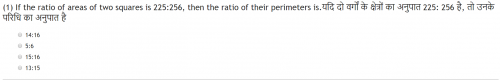# ☆ If the ratio of areas of two squares is 225:256, then the ratio of their perimeters is?

19/Sep/2018, 05:22 AMIf the ratio of areas of two squares is 225:256, then the ratio of their perimeters is.यदि दो वर्गों के क्षेत्रों का अनुपात 225: 256 है, तो उनके परिधि का अनुपात है